## Abstract

Ultra High Performance Strain Hardening Cementitious Composites (UHP-SHCC) are useful for strengthening or repairing concrete members. However there is a need to use refined analytical tools to simulate response of strengthened system. In this study, ABAQUS finite element program is used to numerically perform a parametric study including two major groups. Each group contains nine specimens strengthened from the tension side with variable thickness of UHP-SHCC and reinforced with variable reinforcement ratios embedded in the strengthening layer. Two types of loading were applied: monotonic loading for the first group and cyclic loading for the second group. ABAQUS CPS4R mesh element nonlinear is used to model the concrete, while truss element nonlinear is used to model longitudinal and transverse steel reinforcement. The numerical results obtained are in good agreement with the experimental work found in the literature. The results from the parametric study showed that it is sufficient to use 1.2% additional reinforcement ratio embedded in the strengthening layer for beams strengthened with UHP-SHCC to eliminate the observed early strain localization and to gain adequate ductility under both static and cyclic loadings.

## Keywords

RC beams; Strengthening; Finite-element; UHP-SHCC; Ductility; Repeated load

## 1. Introduction

Various methods are developed for strengthening reinforced concrete beams against flexure. Nowadays, in order to overcome the various shortages of material developed for repairing or retrofitting, high performance cementitious materials are developed for this purpose, which can be called as High Performance Fiber Reinforced Cementitious Composites (HPFRCC) . HPFRCC are normally adopted due to some advantages, such as strain-hardening behavior. One typical type of HPFRCC is strain hardening cementitious composites (SHCC) with higher ductility, which is an attractive material for surface repairing and strengthening. The typical type of SHCC is engineered cementitious composites (ECC) , ,  and . ECC can exhibit tensile strain capacity more than 1% or 2%. However, the durability of ECC may be decreased due to the low performance of matrix . Besides, the compressive strength of ECC is limited. Another typical HPFRCC is Ultra High Performance Fiber Reinforced Concretes (UHPFRC), which exhibit strain-hardening under uniaxial tension and very high strength. However, it also has a limitation of strain capacity.

In order to improve the performance of ordinary HPFRCC, a new type of HPFRCC material, called as Ultra High Performance Strain Hardening Cementitious Composites (UHP-SHCC), is developed by Kunieda et al. , with special objective of high tensile and compressive strength, large strain capacity, high workability, easy processing using conventional equipment, and lower fiber volume fraction. Kunieda et al.  show the target of UHP-SHCC, which has relatively higher hardening strain compared with ordinary UHPFRC, and relatively higher stress than ordinary SHCC. In addition, UHP-SHCC considered a perfect protective material against corrosion . Although having the excellent properties, Ultra High Performance Strain Hardening Cementitious Composites (UHP-SHCC) are not widely adopted in construction until now. One of the primary obstacles preventing the widespread use of UHP-SHCC is the early strain localization occurred in the strengthening layer; therefore, Hussein et al.  presented the ductility behavior in tests on reinforced concrete beams that were strengthened in flexure with lightly steel-reinforced SHCC layer (0.3% and 0.6% steel reinforcement ratio). It has been found that the combination of the SHCC and a small amount of steel reinforcement helps develop higher strain in the SHCC strengthening layer at ultimate load and eliminates the observed early strain localization.

The most reliable method to evaluate accurate behavior of concrete structural elements is to experimentally study actual structures, but because of being expensive and time consuming, usually experiments are not always possible to perform. Therefore, other methods, which take into account the anisotropic behavior of concrete including the effect from tensile cracks, are required. One method is Finite Element Modeling (FEM), which needs less cost and time to be implemented. Different commercial FEM software has been developed during years and one of them is ABAQUS, which was used in this study . ABAQUS is a finite element program used to evaluate the behavior of structures and solids under external loads. This program can analyze both static and dynamic problems and it is capable of modeling a wide range of 2D and 3D shapes and contacts between solids. It has an advanced and extensive library for elements and materials. , proposed a model using finite element analysis that can simulate efficiently the uniaxial tensile behavior of UHP-SHCC, called zero span tensile model which can be useful in this study.

This study concerned on determining numerically the sufficient amount of reinforcement ratio used in UHP-SHCC layer to get the most possible benefit from using this material as a strengthening layer. Although, since the appearance of UHP-SHCC, it has been an important subject of academic research, it mostly focused on understanding the material microstructure and the ductility enhancement under ordinary monotonic loading. However, for design purposes, its structural behavior under repeated loading needs to be thoroughly understood.

## 2. Numerical program

In this section, our objective was to examine the capability of the ABAQUS software  to predict efficiently the static and repeated response of the tested specimens. ABAQUS is a finite element program used to evaluate the behavior of structures and solids under external loads. ABAQUS is one of the most trustworthy FEM programs and different industries including the aircraft and automobile manufacturing industry, microelectronics, oil industry and many research universities and institutes are using this program. ABAQUS has been developed by Hibbitt, Karlsson and Sorensen, Inc. (HKS) through their company established in 1978 .

### 2.1. Material properties and constitutive models

#### 2.1.1. Concrete

In this study, the chosen material model for analyzing concrete in ABAQUS is the concrete damage plasticity model which is compatible with the experimental work found in literature survey due to the following reasons:

• Provides a general capability for modeling concrete and other quasi-brittle materials in all types of structures (beams, trusses, shells, and solids).
• Uses concepts of isotropic damaged elasticity in combination with isotropic tensile and compressive plasticity to represent the inelastic behavior of concrete.
• Designed for applications in which concrete is subjected to monotonic, cyclic, and/or dynamic loading under low confining pressures.

The model assumes that the uniaxial tensile and compressive response of concrete is characterized by damaged plasticity, as shown in Fig. 1. To facilitate the uniaxial tension response of concrete, Fig. 2 shows the stress–strain relationship divided into two parts: first one, shows the behavior up to ultimate tension load which can be considered linear elastic before cracking and described by the elastic modulus (Ec) and tensile strength (fct), and in second part the post-peak behavior can be considered bilinear as shown in Fig. 2. To specify the post-peak tension failure behavior of concrete, the fracture energy method was used. The fracture energy (Gf), is the area under the softening curve. Fig. 3 shows the proposed uniaxial compressive stress–strain curve for concrete obtained from the experimental work. Poisson’s ratio was assumed to be 0.2. As shown in Fig. 1, when the concrete specimen is unloaded from any point on the strain softening branch of the stress–strain curves, the unloading response is weakened: the elastic stiffness of the material appears to be damaged (or degraded). The degradation of the elastic stiffness is characterized by two damage variables, dt and dc, which are assumed to be functions of the plastic strains, temperature, and field variables:

 $d_{t}=d_{t}({\epsilon }_{t}^{\approx {\mbox{pl}}}{\mbox{,}}\theta {\mbox{,}}f_{i}):0\leqslant d_{t}\leqslant 1$
 $d_{c}=d_{c}({\epsilon }_{c}^{\approx {\mbox{pl}}}{\mbox{,}}\theta {\mbox{,}}f_{i}):0\leqslant d_{c}\leqslant 1$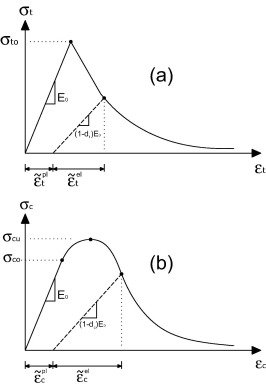Figure 1. Response of concrete to uniaxial loading in tension (a) and compression (b), ABAQUS Manual.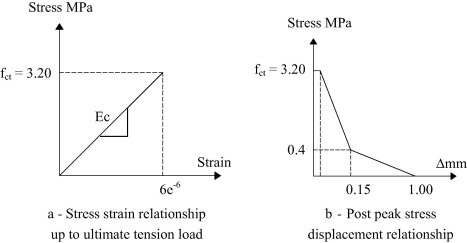Figure 2. Proposed concrete stress–strain relationship under uniaxial tension.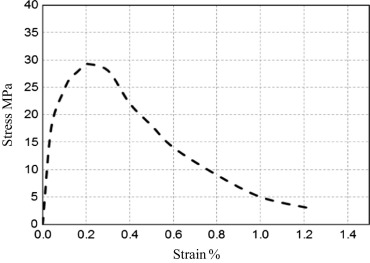Figure 3. Proposed concrete compressive stress–strain relationship.

The damage variables can take values from zero, representing the undamaged material, to one, which represents total loss of strength. If E0 is the initial (undamaged) elastic stiffness of the material, the stress–strain relations under uniaxial tension and compression loading are, respectively:

 ${\sigma }_{t}=(1-d_{t})E_{0}({\epsilon }_{t}-{\epsilon }_{t}^{\approx pl})$
 ${\sigma }_{c}=(1-d_{c})E_{0}({\epsilon }_{c}-{\epsilon }_{c}^{\approx pl})$

#### 2.1.2. Reinforcement

In ABAQUS, reinforcement can be modeled with different methods including smeared reinforcement in the concrete, cohesive element method, discrete truss or beam elements with the embedded region constraint or built-in rebar layers . Rebar defines the uniaxial reinforcement levels in membrane, shell and surface elements. One or multiple layers of reinforcements can be defined and for each layer the rebar layer name, the cross-sectional area of each reinforcement layer and the rebar spacing in the plane of definition should be determined . In this part, just embedded region modeling, which is used for reinforcement modeling in this study, will be explained. Truss element is a common way of reinforcement modeling of which the only required input is the cross-sectional area of bars. Beam element modeling is another common way, which takes into account the dowel effect and increases slightly the load bearing capacity of structures but its use is not recommended because it requires a large number of input parameters to be defined and consequently a high computational effort . According to Hibbit et al.  the effect of bond slip is not considered in the embedded region modeling method but this effect is considered somewhat by definition of the tension stiffening behavior of concrete . The required input parameters for material definition of steel bars, include density, elastic and plastic behavior. Elastic behavior of steel material is defined by specifying Young’s modulus (Es) and Poisson’s ratio (ν) of which typical values are 200 GPa and 0.3, respectively. Plastic behavior is defined in a tabular form, included yield stress and corresponding plastic strain. The steel is assumed to be bilinear elastic–plastic material with stress strain curve as shown in Fig. 4.Figure 4. Stress–strain curve for steel elements.

#### 2.1.3. UHP-SHCC

Zhang  proposed a new type model that can simulate efficiently the uniaxial tensile behavior of UHP-SHCC, called zero span tensile model. The uniaxial stress strain curve obtained experimentally for UHP-SHCC cannot model exactly the behavior of UHP-SHCC layer when attached with concrete substrate because of the strain localization occurred in UHP-SHCC layer in the region of concrete substrate cracks, as described before. This proposed zero-span tensile model is shown in Fig. 5, which also assumed as trilinear curve model considering fracture energy. Points A, B and C for trilinear curve model are the positions corresponding to stress with initial crack, peak stress and minimum stress about zero, based on zero-span tension model, and parameters of stress and strain for points A, B and C are listed in Table 1. The stress and strain of point A are the same with those of material model under uniaxial tensile behavior, due to stress just generates initial crack. The stress of point B is defined by the maximum stress obtained from zero-span tensile behavior, and the strain is defined by the displacement (δB) corresponding to the maximum stress dividing localized area length (Lloc), which is defined as the length of localized strain area. The strain at point C is defined by the fracture energy Gf after peak, which equals to the area of stress–displacement relationship after peak. That is,

 ${\epsilon }_{C}={\epsilon }_{B}+{\frac {2G_{f}}{{\sigma }_{B}\cdot L_{\mbox{elm}}}}$

where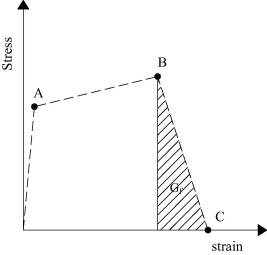Figure 5. Stress–strain curve for zero span tensile model.

Table 1. UHP-SHCC properties input in ABAQUS .
Point A Point B Point C
σ (MPa) ε σ (MPa) ε σ (MPa) ε
4.4 0.00018 5.5 0.011 0 0.17
• εC: strain at point C,
• εB: strain at point B,
• σB: stress at point B,
• Lelm: element size (mm), and
• Gf: the absorbed energy after peak (N/mm).

### 2.2. Interaction between UHP-SHCC and concrete substrate

In order to represent the interaction between the two layers in ABAQUS, two different ways have been used according to the fact that, the solution is two-dimensional or three-dimensional, and also depends on the element used in the model. The first way is to use a surface to surface contact interaction, while the other is to use the node to node contact element. A surface-to-surface contact definition can be used to model contact interactions between specific two surfaces in a model, the master surface and the slave surface. Experimental work has shown that under vertical forces, perfect bond between the UHP-SHCC layer and the concrete substrate was found; therefore, no expected debonding failure may occur.

### 2.3. Boundary conditions and limitations

The actual beam is a three-dimensional, but modeling in 2D space gives in this case the same results with high accuracy and less required time and computational capacity. In order to save the CPU and time of analysis, only half of the beam has been modeled because of symmetrical condition by introducing a symmetry boundary condition along the vertical symmetric-axis of the beam. A steel loading plate and a support plate have been tied up with the concrete beam to remove the stress concentrations around the points of loading and support. All the reinforcements have been modeled with truss elements according to their respective yield or rupture strengths and they are constrained in the concrete by use of embedded region constraint in ABAQUS, which allows each reinforcement element node to connect properly to the nearest concrete node as shown in Fig. 6. This type of bonding does not include the slip effects of reinforcements from concrete beam and instead, these effects were partly considered through the definition of the concrete tension softening. The beams are modeled based on quadrilateral plane stress elements. Mesh elements consist of a four node element with reduced integration function known as CPS4R with an element mesh size of 20 mm. Fig. 6 shows the geometry and boundary conditions of the concrete beam model, meshed as aforesaid.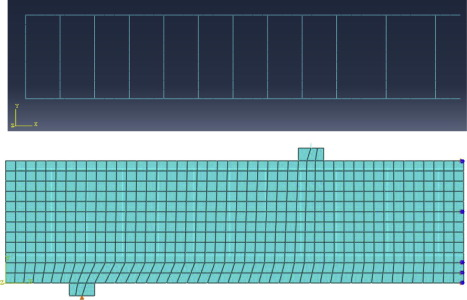Figure 6. Geometry of the model meshed with CPS4R element and Reinforcement modeled as a truss element in ABAQUS.

## 3. Verification against previous experimental work

In order to verify the predictions of the computational model under static loading, the experimental work by Hussein et al.  was considered. Hussein tested experimentally six specimens with dimensions of 200 × 200 × 1800 mm, one control in addition to two specimens strengthened from the soffit side with reinforced mortar and others strengthened with reinforced and unreinforced UHP-SHCC layer. In this verification, only three specimens were chosen: the control one in addition to others strengthened with 0.60% reinforced and unreinforced UHP-SHCC strengthening layer. Three parameters have been pinpointed including the ultimate load, the ultimate deflection and the ductility index. The finite-element predictions are given in Table 2 and Fig. 7 as compared with the reported experimental data of Hussein et al., which were noticed to be in close agreement.

Table 2. Results of verification of numerical analysis against previous work.
Specimen Dimensions (mm) Ultimate load (kN) Ultimate deflection (mm) Ductility index
Finite-element analysis Experimental data (previous work) Finite-element analysis Experimental data (previous work) Finite-element analysis Experimental data (previous work)
Hussein et al.
BC 200 ∗ 200 ∗ 1800 45.3 49.0 38.33 40.15 9.31 11.47
BU0 200 ∗ 200 ∗ 1800 78.0 82.3 5.75 4.77 1.46 1.22
BU2 200 ∗ 200 ∗ 1800 93.5 100.7 11.50 11.00 2.76 2.35
Antony et al.
CB1 120 ∗ 200 ∗ 3200 44.4 41.0 19.35 16.20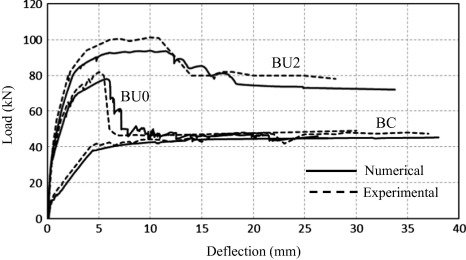Figure 7. Finite-element predictions for .

On the other hand, in order to verify the predictions of the computational model under cyclic loading, the experimental work by Antony et al.  was considered. Antony tested total ten beams over an effective span of 3000 mm and cross-sectional dimensions 125 × 250 mm up to failure under monotonic and cyclic loads. The beams were designed as under-reinforced concrete beams. Eight beams were strengthened with bonded CFRP fabric in single layer and two layers, which are parallel to beam axis at the bottom under virgin condition and tested until failure; the remaining two beams were used as control beams. In this verification, only the control specimen was chosen. Two parameters have been pinpointed including the ultimate load and the ultimate deflection. The finite-element predictions are given in Table 2 and Fig. 8 as compared with the reported experimental data of Antony et al., which were noticed to be in close agreement.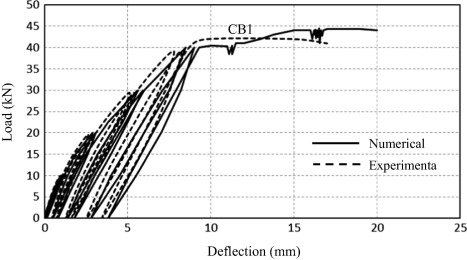Figure 8. Finite-element predictions for .

## 4. Parametric study

Table 3. Description of specimens in Group (I) and Group (II).
Group name Specimen code Dimensions (mm) (L × b × t) Loading type Thickness of UHP-SHCC layer (mm) RFT ratio (%)
Group (I) BS20,0.6% 1800 × 120 × 200 Static 20 0.60
BS40,0.6% Static 40 0.60
BS60,0.6% Static 60 0.60
BS20,1.2% Static 20 1.20
BS40,1.2% Static 40 1.20
BS60,1.2% Static 60 1.20
BS20,1.8% Static 20 1.80
BS40,1.8% Static 40 1.80
BS60,1.8% Static 60 1.80
Group (II) BR20,0.6% Cyclic 20 0.60
BR40,0.6% Cyclic 40 0.60
BR60,0.6% Cyclic 60 0.60
BR20,1.2% Cyclic 20 1.20
BR40,1.2% Cyclic 40 1.20
BR60,1.2% Cyclic 60 1.20
BR20,1.8% Cyclic 20 1.80
BR40,1.8% Cyclic 40 1.80
BR60,1.8% Cyclic 60 1.80

### 4.1. Numerical results and discussions of Group (I)

#### 4.1.1. Load deflection behavior, ductility index and toughness results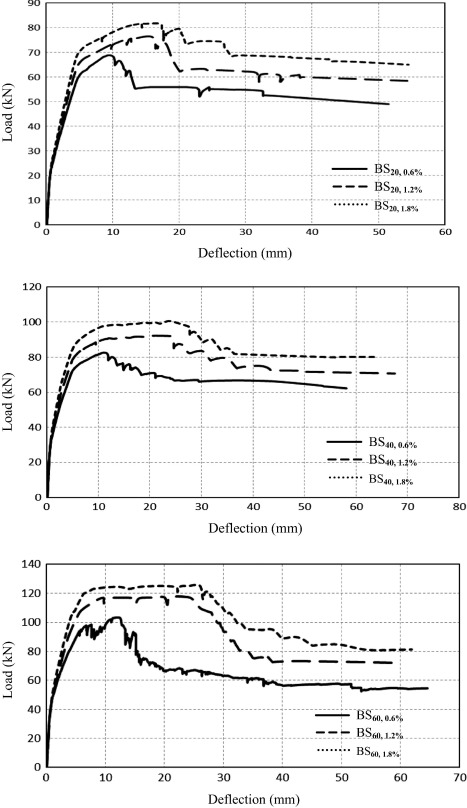Figure 9. Load–deflection relationships for Group (I).

Table 4 lists the values of the ductility indices for all beams in group (I). The higher ductility index ensures that the internal reinforcement experiences plastic deformation in order to provide the desired warning prior to failure of the member. The proposed steel reinforcement enhanced the post-cracking behavior of UHP-SHCC provides a much more ductile behavior for the steel reinforced UHPSHCC strengthening technique. The results shown in Table 4 seem to indicate that the use of steel reinforcement can successfully enhance the ductility of UHP-SHCC strengthened beams. Moreover, 1.8% steel reinforcement enabled beams to attain a ductility index (μΔ) close to ductility index (μΔ) obtained by 1.2% steel reinforcement beams. In addition, toughness was measured as the area under the load–deflection curve up to the peak for each beam, which may be considered as a ductility indicator. As shown in Table 4, the results seem to indicate that the use of steel reinforcement can successfully enhance the ductility of UHP-SHCC strengthened beams. Moreover, 1.8% steel reinforcement enabled beams to attain toughness closer to other obtained by 1.2% steel reinforcement beams.

Table 4. Results of group (I).
Specimen code Yield Ultimate Ductility index Toughness (kN mm)
BS20,0.6% 62.5 5.80 68.8 9.60 1.85 492
BS20,1.2% 67.5 5.50 76.4 15.70 2.85 1098
BS20,1.8% 72.0 5.70 81.7 16.90 2.96 1201
BS40,0.6% 75.1 6.20 82.6 11.80 1.90 965
BS40,1.2% 84.2 6.20 92.2 24.20 3.93 2001
BS40,1.8% 91.3 6.15 100.6 24.40 3.97 2128
BS60,0.6% 93.7 5.90 103.0 12.50 2.12 1237
BS60,1.2% 108.0 6.00 117.3 24.00 4.00 2537
BS60,1.8% 119.0 6.20 125.2 26.00 4.19 2662

#### 4.1.2. Effect of changing additional RFT ratio and thickness of UHP-SHCC layer

Summarizing the previous data, Fig. 10 describes the effect of using variable reinforcement ratios embedded in variable thickness of UHP-SHCC strengthening layer in each of the load carrying capacity, ductility index and toughness for all beams in group (I). From Fig. 10, it can be clearly seen the increasing in load carrying capacity by increasing the strengthening layer thickness and increasing the additional reinforcement ratio embedded in the strengthening layer. Fig. 10 shows that for beams strengthened with 20 mm strengthening layer thickness, 1.8% and 1.2% reinforcement ratio enabled beams to get ductility index 80% and 73% higher than value obtained by 0.6% reinforcement ratio, respectively. In addition, beams strengthened with 40 mm strengthening layer thickness, 1.8% and 1.2% reinforcement ratio enabled beams to get ductility index 105% and 108% higher than value obtained by 0.6% reinforcement ratio, respectively. The same trend obtained by beams strengthened with 60 mm strengthening layer thickness, in which 1.8% and 1.2% reinforcement ratio enabled beams to get ductility index 90% and 100% higher than value obtained by 0.6% reinforcement ratio, respectively.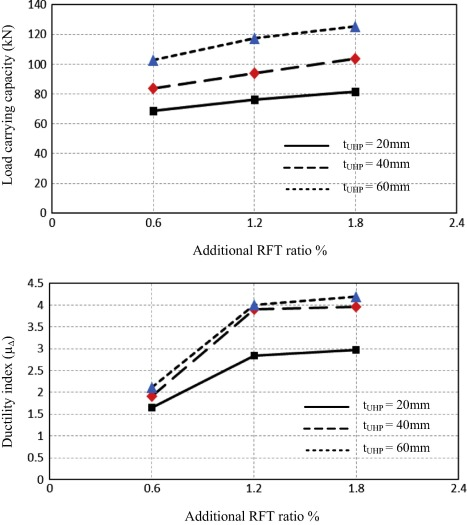Figure 10. Effect of changing additional RFT ratio and thickness of UHP-SHCC layer for group (I).

Generally, it can be clearly noticed the great enhancement in the ductility index values obtained by beams strengthened with 1.2% reinforcement ratio for all different strengthening layer thicknesses, which provides the desired warning prior to failure of the member. However, no great enhancement can be noticed for the ductility index values obtained by beams with 1.8% additional reinforcement ratio compared to beams with 1.2% additional reinforcement ratio. Therefore, it is considered sufficient proof of the insignificant use of reinforcement ratio higher than 1.2% in the ductility enhancement of the strengthened beams. In addition, it can be noticed the convergent values of the ductility index for beams strengthened with 40 mm and 60 mm strengthening layer thickness at each additional reinforcement ratio, which refers to an important conclusion that the desired enhancement in the ductility index values can be obtained by increasing the UHP-SHCC layer thickness up to 40 mm (0.20 of the beam thickness) and increasing thickness over this ratio may lead to insignificant ductility index enhancement.

### 4.2. Numerical results and discussion of Group (II)

#### 4.2.1. Load deflection hysteresis loops and dissipated energy results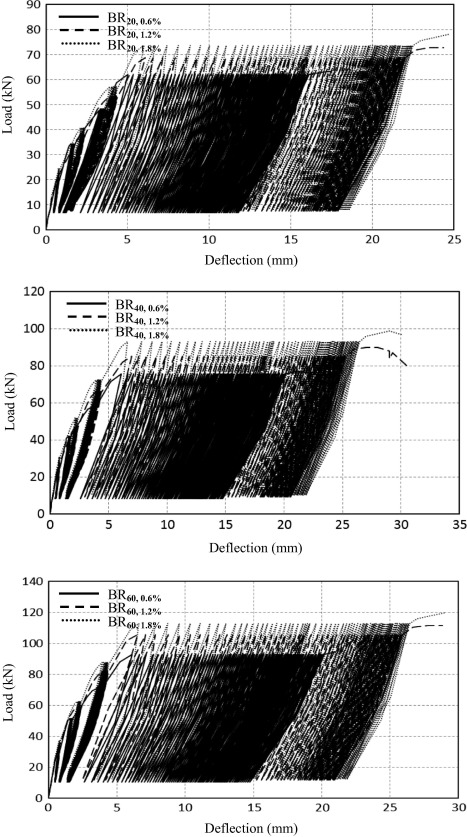Figure 11. Load–deflection hysteresis loops for Group (II).

The dissipated energy is the area enclosed by the hysteresis loop and represents the structural element capacity to mitigate the repeated loading effect which causes excessive cracking and permanent deformation. In UHP-SHCC strengthening layer, fibers inside the matrix also act as energy dissipater because inelastic behavior due to fiber deformation, fiber slip and fiber breaking or pulling out are also main factors along with inelastic behavior of steel bars and cracking of concrete to dissipate major part of the injected energy into the structures during seismic excitation or repeated loads. The energy dissipated during each loading cycle was calculated using the trapezoidal rule to determine the area within the load–deflection hysteresis loop. The total value of dissipated energy in all cycles was determined and is shown in Fig. 12. It can be seen from Fig. 12, the total dissipated energy increased by increasing the additional reinforcement ratio up to 1.2%, and beyond this ratio the increasing in the dissipated energy may be insignificant.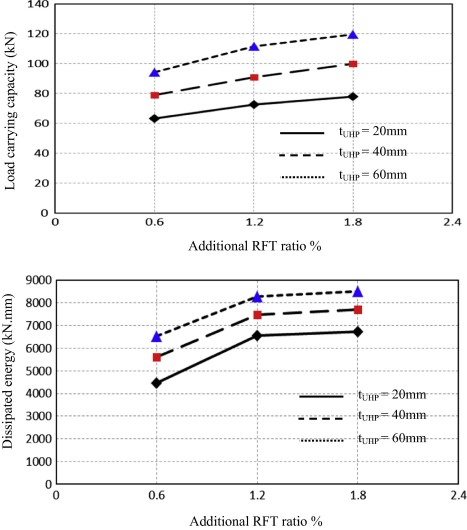Figure 12. Effect of changing additional RFT ratio and thickness of UHP-SHCC layer for group (II).

It is important to know that, from the observation of the hysteresis loops, the energy dissipated in the first cycles of each load level was greater than in the subsequent cycles. This is because that the crack development, widening or propagation occurred in the first cycles while, in the subsequent cycles, the cracks marginally extended in their length but open and close in alternate loading. The possible explanation of greater value of dissipated energy in the first cycles of loading is that when deflection increased, crack extended and the fibers present in the path of the crack resist their propagation causing much energy dissipation. Due to high stress level occurred suddenly in the first cycles, the fibers break during that cycles and no more or little damage of fibers occurs in subsequent cycles of loading at the same load value.

#### 4.2.2. Effect of changing additional RFT ratio and thickness of UHP-SHCC layer

Summarizing the previous data, Fig. 12 describes the effect of using variable reinforcement ratios embedded in variable thickness of UHP-SHCC strengthening layer in each of the load carrying capacity and total dissipated energy for all beams in group (II). From Fig. 12, it can be clearly seen the increasing in load carrying capacity by increasing the strengthening layer thickness and increasing the additional reinforcement ratio embedded in the strengthening layer. Fig. 12 shows that for beams strengthened with 20 mm strengthening layer thickness, 1.8% and 1.2% reinforcement ratio enabled beams to dissipate energy of 52% and 48% higher than value dissipated by beam strengthened with 0.6% reinforcement ratio, respectively. In addition, beams strengthened with 40 mm strengthening layer thickness, 1.8% and 1.2% reinforcement ratio enabled beams to dissipate energy of 38% and 34% higher than value dissipated by beam strengthened with 0.6% reinforcement ratio, respectively. The same trend was obtained by beams strengthened with 60 mm strengthening layer thickness, in which 1.8% and 1.2% reinforcement ratio enabled beams to dissipate energy of 31% and 28% higher than value dissipated by beam strengthened with 0.6% reinforcement ratio, respectively.

In generally, it can be clearly noticed the great dissipated energy values obtained by beams strengthened with 1.2% reinforcement ratio for all different strengthening layer thicknesses. However, no great values of dissipated energy are recorded for beams with 1.8% additional reinforcement ratio compared to beams with 1.2% additional reinforcement ratio. Therefore, it is considered sufficient proof of the insignificant use of reinforcement ratio higher than 1.2% in the ductility enhancement of the strengthened beams subjected to repeated loading.

## 5. Analytical study

### 5.1. Introduction

This section is a trial to estimate flexural capacity of RC beams strengthened with strain hardening cementitious composites. The adopted theoretical approach is verified by comparison with the numerical results. Designing structural members using the ultimate limit state concept is based on the following assumptions:

• Linear strain distribution is assumed. This is based on Bernoulli’s hypothesis that plane sections before bending remain plane and perpendicular to the neutral axis after bending.
• Tensile strength of concrete is neglected. This assumption is made for simplification, since, in fact, concrete resists tension before cracking and after cracking in segments between cracks.
• Full composite action between UHP-SHCC layer and embedded additional reinforcement.
• Perfect bond exists between UHP-SHCC layer and the surrounding concrete.

### 5.2. ACI flexural capacity equation

General equation for the calculation of nominal flexural strength of beam sections strengthened with external FRP attached in the tension side for simple beams with tension steel only is represented  as the following:

 $M_{n}=A_{s}f_{s}\left(d_{s}-{\frac {\lambda c}{2}}\right)+{\psi }_{f}A_{f}f_{fe}\left(d_{f}-\right.$$\left.{\frac {\lambda c}{2}}\right)$

where

• df = distance from extreme compression fiber to the neutral axis of FRP (mm).
• ds = distance from extreme compression fiber to the neutral axis of RFT (mm).
• As = the area of main steel reinforcement (mm2).
• Af = area of FRP external reinforcement (mm2).
• c = distance from extreme compression fiber to the neutral axis (mm).
• ${\textstyle \lambda }$ = ratio of the depth of the equivalent rectangular stress block to the depth of the neutral axis.
• ψf = additional FRP strength-reduction factor.
• fs = stress in tension steel reinforcement (Mpa).
• ${\textstyle f_{fe}}$ = the effective stress in FRP (MPa).

### 5.3. Proposed estimation of flexural capacity of a concrete beam strengthened with UHP-SHCC layer

For beams strengthened with UHP-SHCC layer, ACI 440.2R-02  equations are adopted and modified to take into consideration the effect of compression steel, UHP-SHCC layer and embedded additional reinforcement. General scheme for the internal strain and stress of a rectangular section according to the proposed analysis may be drawn considering the equilibrium of the section under the forces as shown in Fig. 13.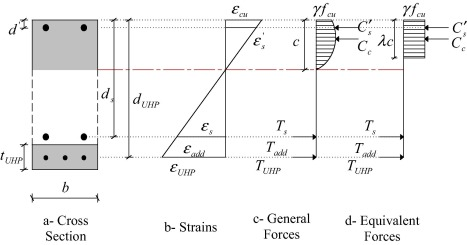Figure 13. Analysis of RC beams.

The equilibrium equation of the section

 $C_{c}+C_{s}^{'}=T_{s}+T_{\mbox{UHP}}+T_{\mbox{add}}$

The nominal moment of the section is calculated by the following equation

 $M_{n}=T_{s}\times \left(d_{s}-{\frac {\lambda c}{2}}\right)+$$T_{\mbox{UHP}}\times \left(d_{\mbox{UHP}}-{\frac {\lambda c}{2}}\right)+$$T_{s{\mbox{,add}}}\times \left(d_{\mbox{UHP}}-{\frac {\lambda c}{2}}\right)+$$C_{s}^{'}\times \left({\frac {\lambda c}{2}}-d^{'}\right)$

where

• Compression force in concrete
 $C_{c}=\gamma \times f_{\mbox{cu}}\times b\times \lambda c$
• Compression force in steel
 $C_{s}^{'}={As}^{'}\times f_{s}^{'}={As}^{'}\times E_{s}{\epsilon }_{\mbox{cu}}{\frac {c-d^{'}}{c}}$
• Tension force in steel
 $T_{s}=A_{s}\times f_{s}=A_{s}\times E_{s}{\epsilon }_{\mbox{cu}}{\frac {d_{s}-c}{c}}$
• Tension force in UHP-SHCC layer
 $T_{\mbox{UHP}}=A_{\mbox{UHP}}\times f_{\mbox{UHP}}=$${bt}_{\mbox{UHP}}\times E_{\mbox{UHP}}{\epsilon }_{\mbox{cu}}{\frac {d_{\mbox{UHP}}-c}{c}}$
• Tension force in additional reinforcement
 $T_{s{\mbox{,add}}}=A_{s{\mbox{,add}}}\times f_{s{\mbox{,add}}}=$$A_{s{\mbox{,add}}}\times E_{s}{\epsilon }_{\mbox{cu}}{\frac {d_{\mbox{UHP}}-c}{c}}$

The flexural nominal moment predictions based on the analytical model are summarized in flowchart as shown in Fig. 14.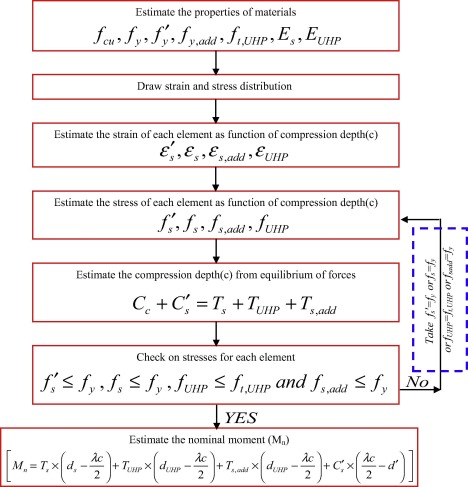Figure 14. Flowchart for nominal moment calculation.

### 5.4. Simplified estimation of flexural capacity of a concrete beam strengthened with UHP-SHCC layer

To simplify the previous proposed equation, only the ductile failure mode is considered and the ratio (d′/d) not exceeds 0.10 according to (ECP 203-2007). That is fs = fy, fs,add = fy,add, fUHP = ft,UHP and fs = fy so the equation may be rewritten as follows:

 $M_{n}=f_{y}A_{s}\left(d_{s}-{\frac {\lambda c}{2}}\right)+f_{t{\mbox{,UHP}}}A_{\mbox{UHP}}\left(d_{\mbox{UHP}}-\right.$$\left.{\frac {\lambda c}{2}}\right)+f_{y{\mbox{,add}}}A_{s{\mbox{,add}}}\times \left(d_{\mbox{UHP}}-\right.$$\left.{\frac {\lambda c}{2}}\right)+f_{y}A_{s}^{'}\times \left({\frac {\lambda c}{2}}-\right.$$\left.d^{'}\right)$

where

 $c={\frac {f_{y}A_{s}+f_{t{\mbox{,UHP}}}A_{\mbox{UHP}}+f_{y{\mbox{,add}}}A_{s{\mbox{,add}}}-f_{y}^{'}A_{s}^{'}}{\gamma \lambda f_{\mbox{cu}}b}}$

### 5.5. Verification of the proposed and simplified model

The proposed equation was adopted for different beams strengthened and tested numerically up to failure; Table 5 shows the values of nominal moment for all beams in group (I) that were calculated by using proposed equation (Mn,proposed), simplified equation (Mn,simplified) and the capacity numerical results (Mn,numerical). Fig. 15 shows a comparison chart between numerical capacity moment versus theoretical values using proposed model and simplified model. From Table 5, it can be concluded that the adopted analytical model succeeded to predict the nominal capacity as close as possible to the numerical investigation. The difference between proposed model results and numerical results ranged between 1% and 11%, with the simplified model results and the difference ranged between 1% and 8%. As a conclusion the adopted and simplified model gave very accurate prediction of the nominal moment for the tested beams in group (I).

Table 5. Verification of proposed and simplified equation for group (I).
Specimen Mn,numerical (kN m) Mn,proposed (kN m) Mn,simplified (kN m) ${\textstyle {\frac {M_{n{\mbox{,numerical}}}}{M_{n{\mbox{,proposed}}}}}}$ ${\textstyle {\frac {M_{n{\mbox{,numerical}}}}{M_{n{\mbox{,simplified}}}}}}$
BS20,0.6% 15.48 14.92 15.18 1.04 1.02
BS20,1.2% 17.19 15.75 16.06 1.09 1.07
BS20,1.8% 18.38 16.58 16.93 1.11 1.08
BS40,0.6% 18.59 18.83 19.28 0.99 0.97
BS40,1.2% 20.75 20.45 20.97 1.01 0.99
BS40,1.8% 22.64 22.05 22.62 1.03 1.00
BS60,0.6% 23.18 22.63 23.22 1.02 0.99
BS60,1.2% 26.39 25.01 25.63 1.05 1.03
BS60,1.8% 28.17 27.25 27.97 1.03 1.01
Average 1.04 1.02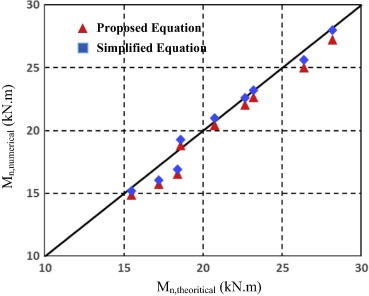Figure 15. Numerical versus theoretical values.

## 6. Conclusions

Based on the results obtained in this work, the following conclusions can be drawn:

• The proposed numerical model in the current study, which was used to represent the behavior of the UHP-SHCC strengthening layer, reinforced concrete beam and reinforcement steel, and was able to predict the actual behavior of the specimens efficiently under both of static and repeated loads.
• Increasing the strengthening layer thickness and increasing the additional reinforcement ratio embedded in the strengthening layer lead to increasing in the load carrying capacities of the specimens subjected to static and repeated loads.
• Beams subjected to static load and strengthened with 1.2% reinforcement ratio embedded in 40 mm UHP-SHCC layer thickness had a great enhancement in the ductility index values, which provide the desired warning prior to failure of the member.
• For beams subjected to static load, increasing UHP-SHCC layer thickness over 40 mm (0.20 of the beam thickness) leads to insignificant ductility index enhancement.
• For beams subjected to static load, increasing the additional reinforcement ratio embedded in the UHP-SHCC strengthening layer over 1.2% leads to insignificant ductility index.
• Increasing the additional reinforcement ratio embedded in the UHP-SHCC strengthening layer over 1.2% leads to insignificant dissipated energy enhancement, for beams subjected to repeated load.
• The adopted and simplified theoretical model gave very accurate prediction of the nominal moment for beams strengthened with UHP-SHCC layer subjected to static load.

### Document informationPublished on 12/04/17

Licence: Other

### Document Score0

Views 67
Recommendations 0